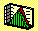2.3 POPULATION STATISTICS

Important properties of the underlying distribution of returns of a security can sometimes be determined by fundamental analysis. Other times they must be inferred from samples and experience. Here, we assume that the financial analyst has identified the ex ante return distribution, but now we want to compute the set of distributional parameters that are relevant to our investment problem.

For simplicity, we look at the discrete random variable case. A similar development applies to the continuous case.

Let x be a random variable. Then, the mean or expected value of x, denoted E(x), is given by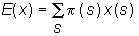.

where p(s) is the probability of state s occurring. When x(s) is the return in state s, then the expected value is the expected return from the security.

The variance of x is given by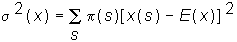The variance provides a measure of scatter, or dispersion, exhibited by the random variable around its mean. The square root of variance is called the standard deviation. When x(s) is a return for some individual security, it is tempting to view the standard deviation of returns as a measure of the security's riskiness. However, as discussed in Chapter 1, topic 1.5, Portfolio Diversification, this overlooks another important property of security returns, which is covariance. An example is seen in the colorful terms that traders use to describe market movements, such as "bull markets" or "bear markets." Traders mean that stocks are on average moving (or co-varying) together.

If we have two random variables, say, x and y, then the covariance of x and y, is given by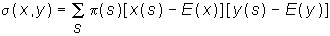where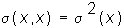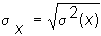,

and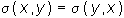.

Formally, covariance provides a measure of the degree to which two random variables move together.

This covariance figure in its raw form can be difficult to interpret because its size depends upon the unit of measurement of x and y. To get around this problem, covariance is often scaled by the product of the two standard deviations to provide the correlation, which is always between -1 and +1. The correlation between x and y, is given by: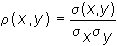Alternatively, population parameters can be estimated from a sample of past prices (see topic 2.4 Sample Statistics).

previous topic

next topic